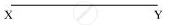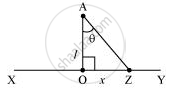Share

# Obtain the Formula for the Electric Field Due to a Long Thin Wire of Uniform Linear Charge Density λ Without Using Gauss’S Law. - CBSE (Science) Class 12 - Physics

#### Question

Obtain the formula for the electric field due to a long thin wire of uniform linear charge density λ without using Gauss’s law. [Hint: Use Coulomb’s law directly and evaluate the necessary integral.]

#### Solution

Take a long thin wire XY (as shown in the figure) of uniform linear charge density lambda .Consider a point A at a perpendicular distance l from the mid-point O of the wire, as shown in the following figure.Let E be the electric field at point A due to the wire, XY.

Consider a small length element dx on the wire section with OZ = x

Let q be the charge on this piece.

therefore q=lambda dx

Electric field due to the piece,

dE=1/(4piin_0)(lambda dx)/((AZ)^2)

However, AZ=sqrt((l^2+x^2))

dE=(lambda dx)/(4piin_0(l^2+x^2))

The electric field is resolved into two rectangular components. dEcostheta is the perpendicular component and  dEsintheta is the parallel component.

When the whole wire is considered, the component dEsintheta is cancelled.

Only the perpendicular component dEcostheta affects point A.

Hence, effective electric field at point A due to the element dx is dE1.

therefore dE_1=(lambdadx costheta)/(4piin_0(x^2+l^2))       ...(1)

In triangleAZO,

tantheta=x/l

x=l tantheta          ...(2)

On differentiating equation (2), we obtain (dx)/(d theta)=lsec^2theta

dx=lsec^2thata dtheta

From equation (2),

x^2+l^2=l^2+l^2 tan theta

therefore l^2(1+tan^2 theta)=l^2sec^2theta

thereforex^2+l^2=l^2sin^2theta     ...(4)

Putting equations (3) and (4) in equation (1), we obtain

dE_1=(lambda l sec^2 d theta)/(4piin_0l^2sec^2 theta)xxcostheta

dE_1=(lambda cos theta d theta)/(4 pi in_0 l)     .......(5)

The wire is so long that theta tends from -pi/2to +pi/2.

By integrating equation (5), we obtain the value of field E1 as,

int_(-pi/2)^(pi/2) dE_1=int_(-pi/2)^(pi/2)lambda/(4piin_0l) cos theta d theta

E_1=lambda/(4piin_0l)[sin theta]_(-pi/2)^(pi/2)

=lambda/(4 pi in_0l)xx2

E_1=lambda/(2piin_0l)

Therefore, the electric field due to long wire islambda/(2piin_0l).

Is there an error in this question or solution?

#### APPEARS IN

NCERT Solution for Physics Textbook for Class 12 (2018 to Current)
Chapter 1: Electric Charge and Fields
Q: 30 | Page no. 49

#### Video TutorialsVIEW ALL 

Solution Obtain the Formula for the Electric Field Due to a Long Thin Wire of Uniform Linear Charge Density λ Without Using Gauss’S Law. Concept: Applications of Gauss’s Law.
S